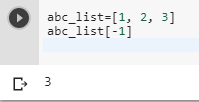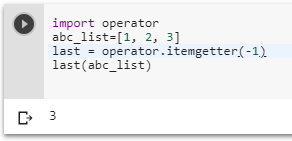2 views
in Python

In Python, how do you get the last element of a list?

closed

by (106k points)
edited by

• We have many ways of getting the last element of the list:-

• The simplest way is to use abc_list[-1]. Let me give you an example that how it works.

abc_list=[1, 2, 3]

abc_list[-1]• The same way you can get all the elements till first using this method.

Syntax: abc_list[-n]

• Where n  = index of an element from last that you want.

• Below is the algorithm to get the first element of the list.

abc_list[-len(some_list)]

Or

abc_list

• But if your list is empty when you perform this method then you will get indexNotFound Error. So make sure your list is not empty when you are trying to get elements of your list by this method.

• The second method to get the last element is to use operator.itemgetter:

import operator

abc_list=[1, 2, 3]

last = operator.itemgetter(-1)

last(abc_list)• You can also do by list slicing a slice of a list returns a new list so we can slice from -1 to the end if we are going to want the element in a new list:-

abc_list=[1, 2,3]

abc_slice = abc_list[-1:]

abc_slice• It has an upper hand that it does not fail if your list is empty. Instead of giving index not found Error like the above method it will return you an empty list.